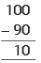# Olympiad Test: Subtraction - 1

## 15 Questions MCQ Test Mathematics Olympiad Class 4 | Olympiad Test: Subtraction - 1

Description
This mock test of Olympiad Test: Subtraction - 1 for Class 4 helps you for every Class 4 entrance exam. This contains 15 Multiple Choice Questions for Class 4 Olympiad Test: Subtraction - 1 (mcq) to study with solutions a complete question bank. The solved questions answers in this Olympiad Test: Subtraction - 1 quiz give you a good mix of easy questions and tough questions. Class 4 students definitely take this Olympiad Test: Subtraction - 1 exercise for a better result in the exam. You can find other Olympiad Test: Subtraction - 1 extra questions, long questions & short questions for Class 4 on EduRev as well by searching above.
QUESTION: 1

Solution:
QUESTION: 2

### The result obtained after subtraction is called -----------

Solution:

The result after subtraction is called difference.

QUESTION: 3

### --------- is the number that is to be subtracted from.

Solution:
QUESTION: 4

Pick the odd one out.

Solution:

Clearly sum is different. Rest all decrease the value of a quantity while sum increases the quantity.

QUESTION: 5

Find the Minuend:
? – 5 = 45

Solution:
QUESTION: 6

When we subtract a number from itself, the answer is always ---------

Solution:

When we subtract a number from itself, the answer is always zero.

QUESTION: 7

Fill in the blank.
115 – ? = 0

Solution:
QUESTION: 8

When we subtract --------- from a number, the answer is the number itself

Solution:
QUESTION: 9

Fill in the blank.
217 – ? = 217

Solution:

Here 217 – ? = 217
⇒ ? = 217 – 217 = 0

QUESTION: 10

Read the statements carefully and choose the correct option.
Statement A : Minuend – Subtrahend = Difference
Statement B : Minuend – Difference =Subtrahend

Solution:
QUESTION: 11

Find the Subtrahend:
100 – ? = 90

Solution:

Here 100 – ? = 90 ⇒ ? = 100 – 90 =10QUESTION: 12

Tick the correct option.
​1. 999 – 0 = 999
2. 999 – 999 =0

Solution:

Here 999 – 0 = 999 and 999 – 999 = 0
Hence both are correct.

QUESTION: 13

Chose the correct option.
1. 812 – 0 = 0
2. 812 – 812 = 0

Solution:
QUESTION: 14

Tick the correct option.
1. Subtraction is used to find out how many are left.
2. Subtraction is used to find out the total amount.

Solution:

Only 1 is correct.

QUESTION: 15

Solve the problem
15 +  ? = 25

Solution:

15 + ? = 25 ⇒ ? = 25 – 15 = 10